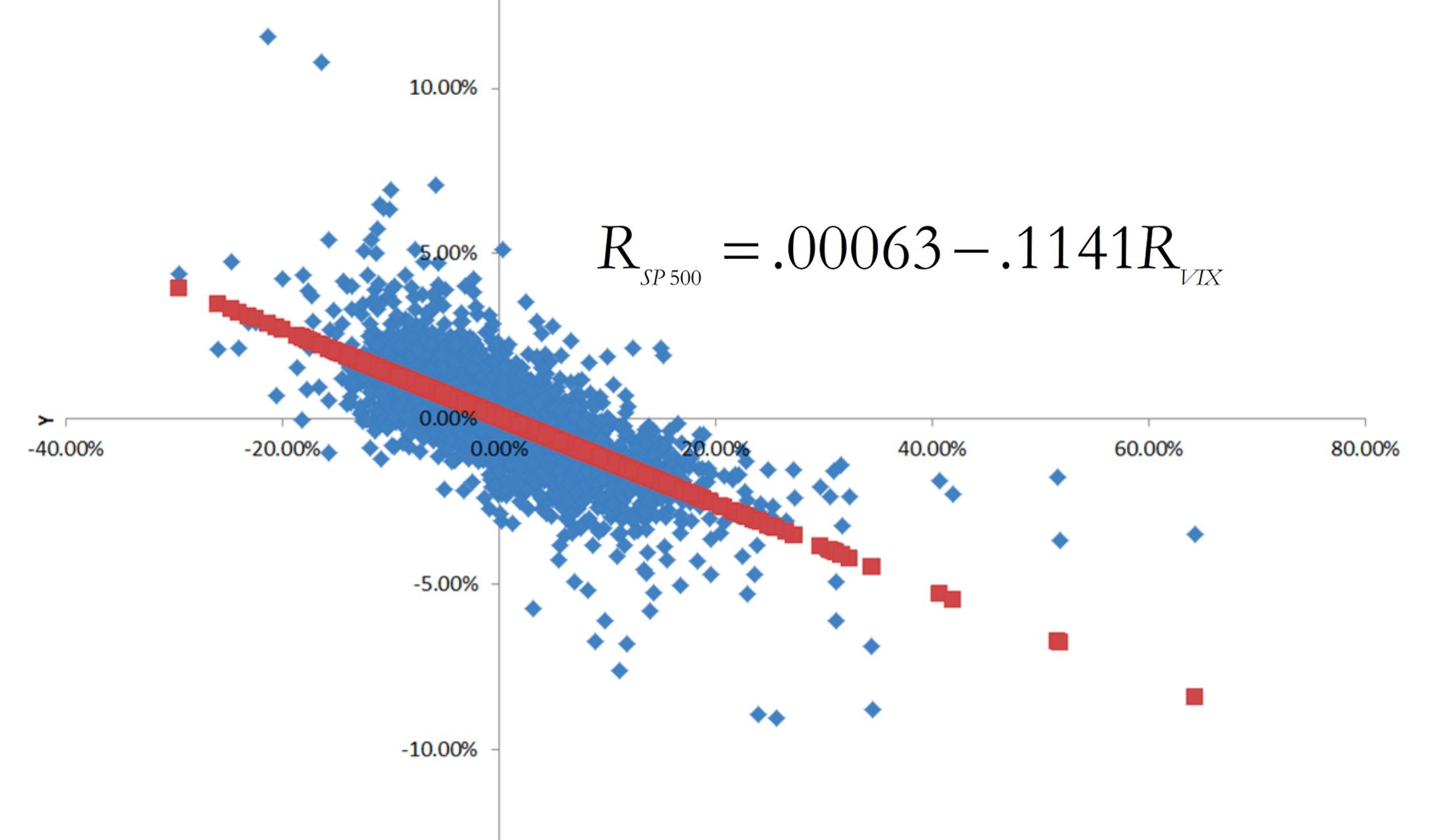## On the relationship between the S&P 500 and the CBOE Volatility Index (VIX)

Besides going over the course syllabus during the first day of class on Tuesday, August 23, we will also discuss a particularly important “real world” example of financial risk. Specifically, we will study the relationship between realized daily stock market returns (as measured by daily percentage changes in the SP500 stock market index) and changes in forward-looking investor expectations of stock market volatility (as indicated by daily percentage changes in the CBOE Volatility Index (VIX)):As indicated by this graph (which also appears in the lecture note for the first day of class), daily percentage changes on closing prices for the SP500 (the y-axis variable) and for the VIX (the x-axis variable) are strongly negatively correlated with each other. The blue dots are based on 8,213 contemporaneous observations of daily returns for both variables, spanning the (more than 33-year) time period from January 2, 1990, through August 5, 2022. When we fit a regression line through this scatter diagram, we obtain the following equation:${R_{SP500}} = .00063 - .1141{R_{VIX}}$,

where${R_{SP500}}$ corresponds to the daily return on the SP500 index and${R_{VIX}}$ corresponds to the daily return on the VIX index. The slope of this line (-0.1141) indicates that on average, daily realized SP500 returns during this time period were inversely related to contemporaneous daily returns on the VIX; i.e., when forward-looking investor expectations of stock market volatility fell (rose), then the stock market return as indicated by SP500 typically rose (fell). Nearly half of the variation in the stock market return during this time period (specifically, 49.12%) can be statistically “explained” by changes in volatility, and the correlation between${R_{SP500}}$ and${R_{VIX}}$ comes out to -0.70. While a correlation of -0.70 does not imply that daily closing values for${R_{SP500}}$ and${R_{VIX}}$ always move in opposite directions, it does suggest that this will be the case more often than not. Indeed, closing daily values recorded for${R_{SP500}}$ and${R_{VIX}}$ during this period moved inversely 78.63% of the time.